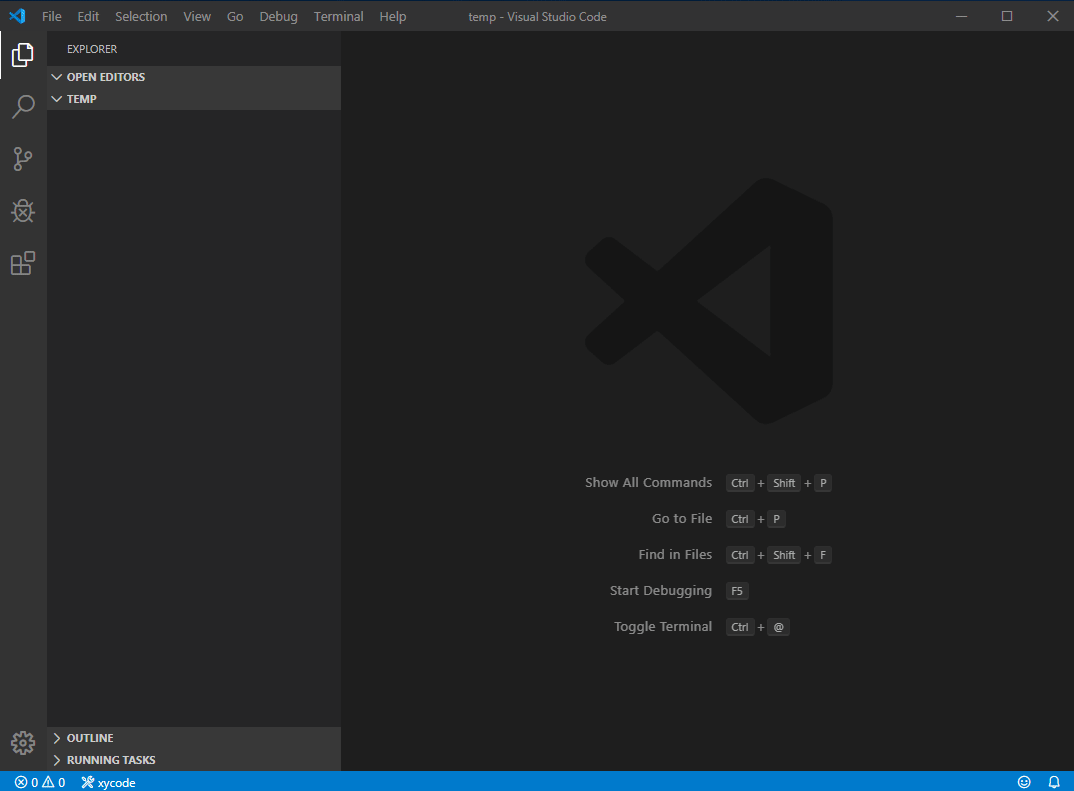# xymath for vscode

Posted by ExiaHuang on January 2, 2020

xymath is a wapper for qalc and gnuplot command. You need to download qalculate and gnuplot if you want to use this plugin.

What is Qalculate ? Qalculate! is a multi-purpose cross-platform desktop calculator. It is simple to use but provides power and versatility normally reserved for complicated math packages.

What is gnuplot ? gnuplot is a portable command-line driven graphing utility.

## Features

• math calculator.
• autorun .qalc file after save.
• autorun .gp .gnuplot file after save.

## usage

### use Qalculate

create a .qalc file, and write some math formula, then save it.

example:

• exchange rates
• Calculus
• change Units### use gnuplot

create a .gp file, and save it.

example:

• plot sin(x)• Qalculate
• gnuplot

## Extension Settings

1
2
3
4
5
{
"xymath.qalculate": "the path of qalculate, default : qalc",
"xymath.gnuplot": "the path of gnuplot, default : gnuplot",
"xymath.encoding": "if encoding error, you can set this value"
}


Chinese user: “xymath.encoding” : “CP936”,

Japanese user: “xymath.encoding” : “CP932”,

Enjoy!